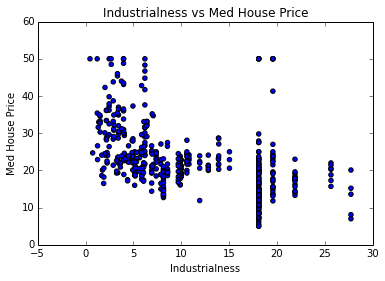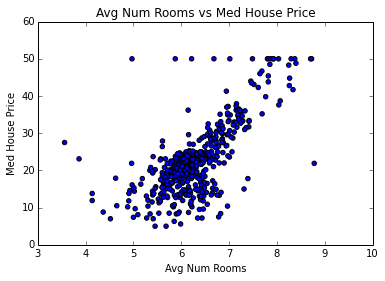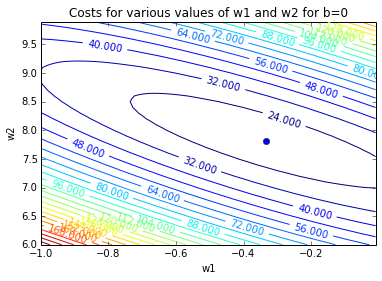# Tutorial: Linear Regression¶

Agenda:

1. Spyder interface
2. Linear regression running example: boston data
3. Vectorize cost function
4. Closed form solution
In :
import matplotlib
import numpy as np
import matplotlib.pyplot as plt
%matplotlib inline

In :
from sklearn.datasets import load_boston
print(boston_data['DESCR'])

Boston House Prices dataset

Notes
------
Data Set Characteristics:

:Number of Instances: 506

:Number of Attributes: 13 numeric/categorical predictive

:Median Value (attribute 14) is usually the target

:Attribute Information (in order):
- CRIM     per capita crime rate by town
- ZN       proportion of residential land zoned for lots over 25,000 sq.ft.
- INDUS    proportion of non-retail business acres per town
- CHAS     Charles River dummy variable (= 1 if tract bounds river; 0 otherwise)
- NOX      nitric oxides concentration (parts per 10 million)
- RM       average number of rooms per dwelling
- AGE      proportion of owner-occupied units built prior to 1940
- DIS      weighted distances to five Boston employment centres
- TAX      full-value property-tax rate per $10,000 - PTRATIO pupil-teacher ratio by town - B 1000(Bk - 0.63)^2 where Bk is the proportion of blacks by town - LSTAT % lower status of the population - MEDV Median value of owner-occupied homes in$1000's

:Missing Attribute Values: None

:Creator: Harrison, D. and Rubinfeld, D.L.

This is a copy of UCI ML housing dataset.
http://archive.ics.uci.edu/ml/datasets/Housing

This dataset was taken from the StatLib library which is maintained at Carnegie Mellon University.

The Boston house-price data of Harrison, D. and Rubinfeld, D.L. 'Hedonic
prices and the demand for clean air', J. Environ. Economics & Management,
vol.5, 81-102, 1978.   Used in Belsley, Kuh & Welsch, 'Regression diagnostics
...', Wiley, 1980.   N.B. Various transformations are used in the table on
pages 244-261 of the latter.

The Boston house-price data has been used in many machine learning papers that address regression
problems.

**References**

- Belsley, Kuh & Welsch, 'Regression diagnostics: Identifying Influential Data and Sources of Collinearity', Wiley, 1980. 244-261.
- Quinlan,R. (1993). Combining Instance-Based and Model-Based Learning. In Proceedings on the Tenth International Conference of Machine Learning, 236-243, University of Massachusetts, Amherst. Morgan Kaufmann.
- many more! (see http://archive.ics.uci.edu/ml/datasets/Housing)


In :
# take the boston data
data = boston_data['data']
# we will only work with two of the features: INDUS and RM
x_input = data[:, [2,5]]
y_target = boston_data['target']

In :
# Individual plots for the two features:
plt.title('Industrialness vs Med House Price')
plt.scatter(x_input[:, 0], y_target)
plt.xlabel('Industrialness')
plt.ylabel('Med House Price')
plt.show()

plt.title('Avg Num Rooms vs Med House Price')
plt.scatter(x_input[:, 1], y_target)
plt.xlabel('Avg Num Rooms')
plt.ylabel('Med House Price')
plt.show()## Define cost function¶

$$\mathcal{E}(y, t) = \frac{1}{2N} \sum_{i=1}^N (y^{(i)}-t^{(i)})^2$$$$\mathcal{E}(y, t) = \frac{1}{2N} \sum_{i=1}^N (w_1 x_1^{(i)} + w_2 x_2^{(i)} + b -t^{(i)})^2$$
In :
def cost(w1, w2, b, X, t):
'''
Evaluate the cost function in a non-vectorized manner for
inputs X and targets t, at weights w1, w2 and b.
'''
costs = 0
for i in range(len(t)):
y_i = w1 * X[i, 0] + w2 * X[i, 1] + b
t_i = t[i]
costs += 0.5 * (y_i - t_i) ** 2
return costs / len(t)

In :
cost(3, 5, 20, x_input, y_target)

Out:
2241.1239166749006
In :
cost(3, 5, 0, x_input, y_target)

Out:
1195.1098850543478

## Vectorizing the cost function:¶

$$\mathcal{E}(y, t) = \frac{1}{2N} \| \bf{X} \bf{w} + b \bf{1} - \bf{t} \| ^2$$

In :
def cost_vectorized(w1, w2, b, X, t):
'''
Evaluate the cost function in a vectorized manner for
inputs X and targets t, at weights w1, w2 and b.
'''
N = len(y_target)
w = np.array([w1, w2])
y = np.dot(X, w) + b * np.ones(N)
return np.sum((y - t)**2) / (2.0 * N)

In :
cost_vectorized(3, 5, 20, x_input, y_target)

Out:
2241.1239166749015
In :
cost(3, 5, 0, x_input, y_target)

Out:
1195.1098850543478

## Comparing speed of the vectorized vs unvectorized code¶

We'll see below that the vectorized code already runs ~2x faster than the non-vectorized code!

Hopefully this will convince you to always vectorized your code whenever possible

In :
import time

t0 = time.time()
print cost(4, 5, 20, x_input, y_target)
t1 = time.time()
print t1 - t0

3182.40634167
0.00229597091675

In :
t0 = time.time()
print cost_vectorized(4, 5, 20, x_input, y_target)
t1 = time.time()
print t1 - t0

3182.40634167
0.000537872314453


## Plotting cost in weight space¶

We'll plot the cost for two of our weights, assuming that bias = -22.89831573.

We'll see where that number comes from later.

Notice the shape of the contours are ovals.

In :
w1s = np.arange(-1.0, 0.0, 0.01)
w2s = np.arange(6.0, 10.0, 0.1)
z_cost = []
for w2 in w2s:
z_cost.append([cost_vectorized(w1, w2, -22.89831573, x_input, y_target) for w1 in w1s])
z_cost = np.array(z_cost)
np.shape(z_cost)
W1, W2 = np.meshgrid(w1s, w2s)
CS = plt.contour(W1, W2, z_cost, 25)
plt.clabel(CS, inline=1, fontsize=10)
plt.title('Costs for various values of w1 and w2 for b=0')
plt.xlabel("w1")
plt.ylabel("w2")
plt.plot([-0.33471389], [7.82205511], 'o') # this will be the minima that we'll find later
plt.show()# Exact Solution¶

Work this out on the board:

2. get equations from partial derivative
3. vectorize
4. write code.
In :
# add an extra feature (column in the input) that are just all ones
x_in = np.concatenate([x_input, np.ones([np.shape(x_input), 1])], axis=1)
x_in

Out:
array([[  2.31 ,   6.575,   1.   ],
[  7.07 ,   6.421,   1.   ],
[  7.07 ,   7.185,   1.   ],
...,
[ 11.93 ,   6.976,   1.   ],
[ 11.93 ,   6.794,   1.   ],
[ 11.93 ,   6.03 ,   1.   ]])
In :
def solve_exactly(X, t):
'''
Solve linear regression exactly. (fully vectorized)

Given X - NxD matrix of inputs
t - target outputs
Returns the optimal weights as a D-dimensional vector
'''
N, D = np.shape(X)
A = np.matmul(X.T, X)
c = np.dot(X.T, t)
return np.matmul(np.linalg.inv(A), c)

In :
solve_exactly(x_in, y_target)

Out:
array([ -0.33471389,   7.82205511, -22.89831573])
In :
# In real life we don't want to code it directly
np.linalg.lstsq(x_in, y_target)

Out:
(array([ -0.33471389,   7.82205511, -22.89831573]),
array([ 19807.614505]),
3,
array([ 318.75354429,   75.21961717,    2.10127199]))

$$\frac{\partial \mathcal{E}}{\partial w_j} = \frac{1}{N}\sum_i x_j^{(i)}(y^{(i)}-t^{(i)})$$
In :
# Vectorized gradient function
'''
Given weights - a current "Guess" of what our weights should be
X - matrix of shape (N,D) of input features
t - target y values
Return gradient of each weight evaluated at the current value
'''
N, D = np.shape(X)
y_pred = np.matmul(X, weights)
error = y_pred - t
return np.matmul(np.transpose(x_in), error) / float(N)

In :
def solve_via_gradient_descent(X, t, print_every=5000,
niter=100000, alpha=0.005):
'''
Given X - matrix of shape (N,D) of input features
t - target y values
Solves for linear regression weights.
Return weights after niter iterations.
'''
N, D = np.shape(X)
# initialize all the weights to zeros
w = np.zeros([D])
for k in range(niter):
w = w - alpha*dw
if k % print_every == 0:
print 'Weight after %d iteration: %s' % (k, str(w))
return w

In :
solve_via_gradient_descent( X=x_in, t=y_target)

Weight after 0 iteration: [ 1.10241186  0.73047508  0.11266403]
Weight after 5000 iteration: [-0.48304613  5.10076868 -3.97899253]
Weight after 10000 iteration: [-0.45397323  5.63413678 -7.6871518 ]
Weight after 15000 iteration: [ -0.43059857   6.06296553 -10.66851736]
Weight after 20000 iteration: [ -0.41180532   6.40774447 -13.06553969]
Weight after 25000 iteration: [ -0.39669551   6.68494726 -14.9927492 ]
Weight after 30000 iteration: [ -0.38454721   6.90781871 -16.54222851]
Weight after 35000 iteration: [ -0.37477995   7.08700769 -17.78801217]
Weight after 40000 iteration: [ -0.36692706   7.23107589 -18.78962409]
Weight after 45000 iteration: [ -0.36061333   7.34690694 -19.59492155]
Weight after 50000 iteration: [ -0.35553708   7.44003528 -20.24238191]
Weight after 55000 iteration: [ -0.35145576   7.5149106  -20.762941  ]
Weight after 60000 iteration: [ -0.34817438   7.57511047 -21.18147127]
Weight after 65000 iteration: [ -0.34553614   7.62351125 -21.51797024]
Weight after 70000 iteration: [ -0.343415     7.66242555 -21.78851591]
Weight after 75000 iteration: [ -0.34170959   7.69371271 -22.00603503]
Weight after 80000 iteration: [ -0.34033844   7.71886763 -22.18092072]
Weight after 85000 iteration: [ -0.33923604   7.73909222 -22.32152908]
Weight after 90000 iteration: [ -0.3383497    7.75535283 -22.4345784 ]
Weight after 95000 iteration: [ -0.33763709   7.76842638 -22.52547023]

Out:
array([ -0.33706425,   7.77893565, -22.59853432])
In :
# For comparison, this was the exact result:
np.linalg.lstsq(x_in, y_target)

Out:
(array([ -0.33471389,   7.82205511, -22.89831573]),
array([ 19807.614505]),
3,
array([ 318.75354429,   75.21961717,    2.10127199]))